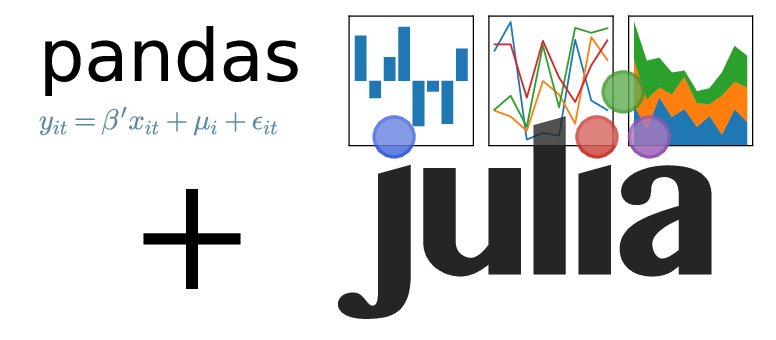101

7

17

10

# Pandas.jlThis package provides a Julia interface to the excellent Pandas package. It sticks closely to the Pandas API. One exception is that integer-based indexing is automatically converted from Python's 0-based indexing to Julia's 1-based indexing.

## Installation

You must have Pandas installed. Usually you can do that on the command line by typing

``````sudo pip install pandas
``````

It also comes with the Anaconda and Enthought Python distributions.

Then in Julia, type

``````Pkg.add("Pandas")
using Pandas
``````

No-hassle installation is also available via Docker:

``````docker run -it malmaud/julia_pandas
``````

## Usage

In general, if `df` is a Pandas object (such as a dataframe or series), then the Python command `df.x(y, w=z)` becomes `x(df, y, w=z)` in Julia. `df.loc[a,b,c]` becomes `loc(df)[a,b,c]` (same for `iloc` and `ix`). Example:

``````>> using Pandas
>> df = DataFrame(Dict(:age=>[27, 29, 27], :name=>["James", "Jill", "Jake"]))
age   name
0   27  James
1   29   Jill
2   27   Jake

[3 rows x 2 columns]
>> describe(df)
age
count   3.000000
mean   27.666667
std     1.154701
min    27.000000
25%    27.000000
50%    27.000000
75%    28.000000
max    29.000000

[8 rows x 1 columns]

df[:age]
0    27
1    29
2    27
Name: age, dtype: int64

>> df2 = DataFrame(Dict(:income=>[45, 101, 87]), index=["Jake", "James", "Jill"])
>> df3 = merge(df, df2, left_on="name", right_index=true)
age   name  income
0   27  James     101
1   29   Jill      87
2   27   Jake      45

[3 rows x 3 columns]

>> iloc(df3)[1:2, 2:3]
name  income
0  James     101
1   Jill      87

[2 rows x 2 columns]

>> mean(groupby(df3, "age")) #Or groupby(df, "age3") |> mean
income
age
27       73
29       87

[2 rows x 1 columns]

>> query(df3, :(income>85)) # or query(df3, "income>85")
age   name  income
0   27  James     101
1   29   Jill      87

[2 rows x 3 columns]

>> Array(df3)
3x3 Array{Any,2}:
27  "James"  101
29  "Jill"    87
27  "Jake"    45

>> plot(df3)
``````

## Input/Output

Example:

``````df = read_csv("my_csv_file.csv") # Read in a CSV file as a dataframe
to_json(df, "my_json_file.json") # Save a dataframe to disk in JSON format
``````

## Performance

Most Pandas operations on medium to large dataframes are very fast, since the overhead of calling into the Python API is small compared to the time spent inside Pandas' highly efficient C implementation.

Setting and getting individual elements of a dataframe or series is slow however, since it requires a round-trip of communication with Python for each operation. Instead, use the `values` method to get a version of a series or homogeneous dataframe that requires no copying and is as fast to access and write to as a Julia native array. Example:

``````>> x_series = Series(randn(10000))
>> @time x_series
elapsed time: 0.000121945 seconds (2644 bytes allocated)
>> x_values = values(x_series)
>> @time x_values
elapsed time: 2.041e-6 seconds (64 bytes allocated)
>> x_native = randn(10000)
>> @time x_native
elapsed time: 2.689e-6 seconds (64 bytes allocated)
``````

Changes to the values(...) array propogate back to the underlying series/dataframe:

``````>> iloc(x_series)
-0.38390854447454037
>> x_values = 10
>> iloc(x_series)
10
``````

## Caveats

Panels-related functions are still unwrapped, as well as a few other obscure functions. Note that even if a function is not wrapped explicitly, it can still be called using various methods from PyCall.

01/24/2014

2 days ago

152 commits